Waves Cheatsheet

Waves

We can categorize waves according to their propagation direction under two title; longitudinal waves and transverse waves.

Transverse Wave: In these types of waves, directions of wave and motion of particles are perpendicular to each other. Picture given below shows this wave type.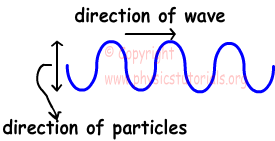Longitudinal Wave: In this type of waves, direction of the particles and wave are same. Look at the given picture below.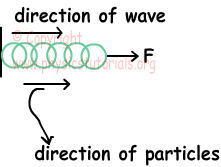Pulse:  one wave motion created at the spring.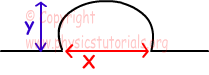Where; x is the pulse length and y is the amplitude (height of the pulse).

Wavelength:It is the distance between two points of two waves having same characteristics.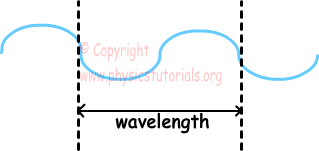Wavelength is shown with the Greek letter "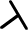" and unit of it is "m".

Period: Time required for production of one wave is called period. It is shown with letter "T" and its unit is "s".

Frequency: It is the number of waves produced in a given unit of time. It is shown with letter "f" and its unit is "1/s".

Periodic Wave: If the wave source produces equal number of waves in equal times, then this wave called periodic wave.

f=1/T

Velocity of the Wave: Velocity of the wave is constant in a given medium. However, if the medium is changed then velocity of the wave is also changed.  We show velocity with "v" and its unit is m/s.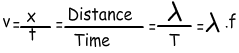Velocity of the Spring Pulse

Distance taken at an instant time by the pulse is called velocity of the pulse. Velocity of the spring pulse depends on force exerted on the spring and spring constant µ. Spring constant depends on the type of the spring; it is found by the following formula;

µ=mass/length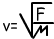Where; v velocity of the spring pulse (m/s), µ is the spring constant (kg/m), and F is the force exerted on the spring (N).

Interference of Spring Waves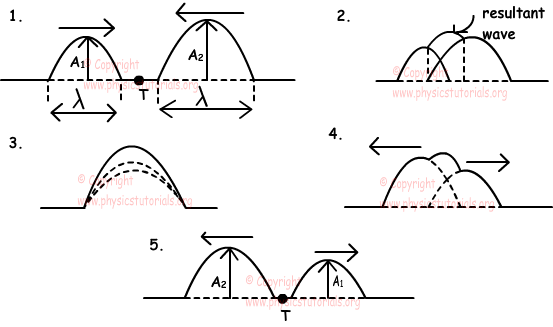Reflection of Spring Waves

a) Reflection from Fixed End:

When a pulse of spring wave hits an obstacle having fixed end, it reflects. Reflected wave has opposite direction, same amplitude and velocity with the incident wave. Picture given below shows this process.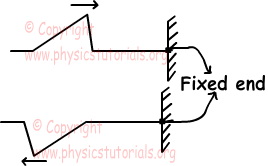b) Reflection from Open End :

When a pulse of a spring comes to an open end obstacle it reflects, like given picture below. Amplitude, velocity and length of the pulse do not change but its right hand side becomes left hand side.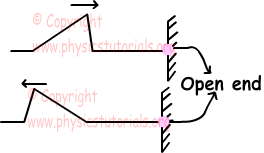Reflection from High Density to Low Density and Low Density to High Density: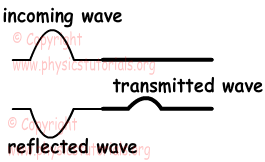Relation between the velocities of incident transmitted and reflected wave;

vincident=vreflected>vtransmitted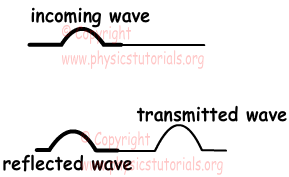Amplitudes of the incident wave and reflected wave are equal but amplitude of transmitted wave is larger than them. Relation between the velocities of incident, reflected and transmitted waves;

vincident=vreflected<vtransmitted

Refraction of Waves

Waves change direction when passing from one medium to another. This change in the direction of wave is called refraction of wave. During refraction velocity and wavelength of waves change however, frequency of waves stay constant. Velocity and wavelength of wave coming from deep part of water tank to shallow part decrease. We can write following equations for incident and refracted waves;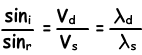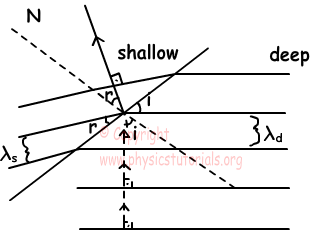Waves Exams and Solutions

Author: